# The IRT Procedure

### Approximating the Marginal Likelihood

Subsections:

As discussed in the section Marginal Likelihood, integrations that are involved in the marginal likelihood for IRT model cannot be solved analytically and need to be approximated by using numerical integration, mostly Gauss-Hermite quadrature.

#### Gauss-Hermite (G-H) Quadrature

In general, the Gauss-Hermite (G-H) quadrature can be presented as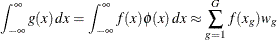where G is the number of quadrature points and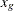and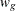are the integration points and weights, respectively, which are uniquely determined by the integration domain and the weighting kernel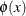. Traditional G-H quadrature often uses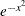as the weighting kernel. In the field of statistics, the density of standard normal distribution is more widely used instead, because for estimating various statistical models, the Gaussian density is often a factor of the integrand. In the case in which the Gaussian density is not a factor of the integrand, the integral is transformed into the form by dividing and multiplying the original integrand by the standard normal density.

#### Adaptive Gauss-Hermite Quadrature

The G order G-H quadrature is exact if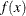is a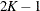degree polynomial in x. However, as many researchers (Lesaffre and Spiessens 2001; Rabe-Hesketh, Skrondal, and Pickles 2002) point out, integrands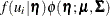often have sharp peaks and cannot be well approximated by low-degree polynomials in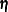. Furthermore, the peak might be far from zero or be located between adjacent quadrature points so that substantial contributions to the integral are lost.

Note that the integrands in the marginal likelihood are a product of the prior density of,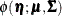and the joint probability of responses given,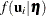. After normalization with respect to, the integrand,, is just the posterior density of, given the observed responses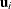. This posterior density is approximately normal when the number of items is large. Let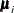and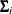be the mean and covariance matrix, respectively, of the posterior density. Then the ratio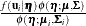can be well approximated by a low-degree polynomial if the number of items is relatively large. This suggests that the integral should be transformed as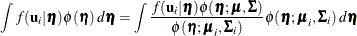The integration points and weights that correspond to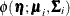are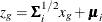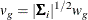The preceding transformations move and scale the quadrature points to the center of the integrands such that the integrand can be better approximated using many fewer quadrature points.